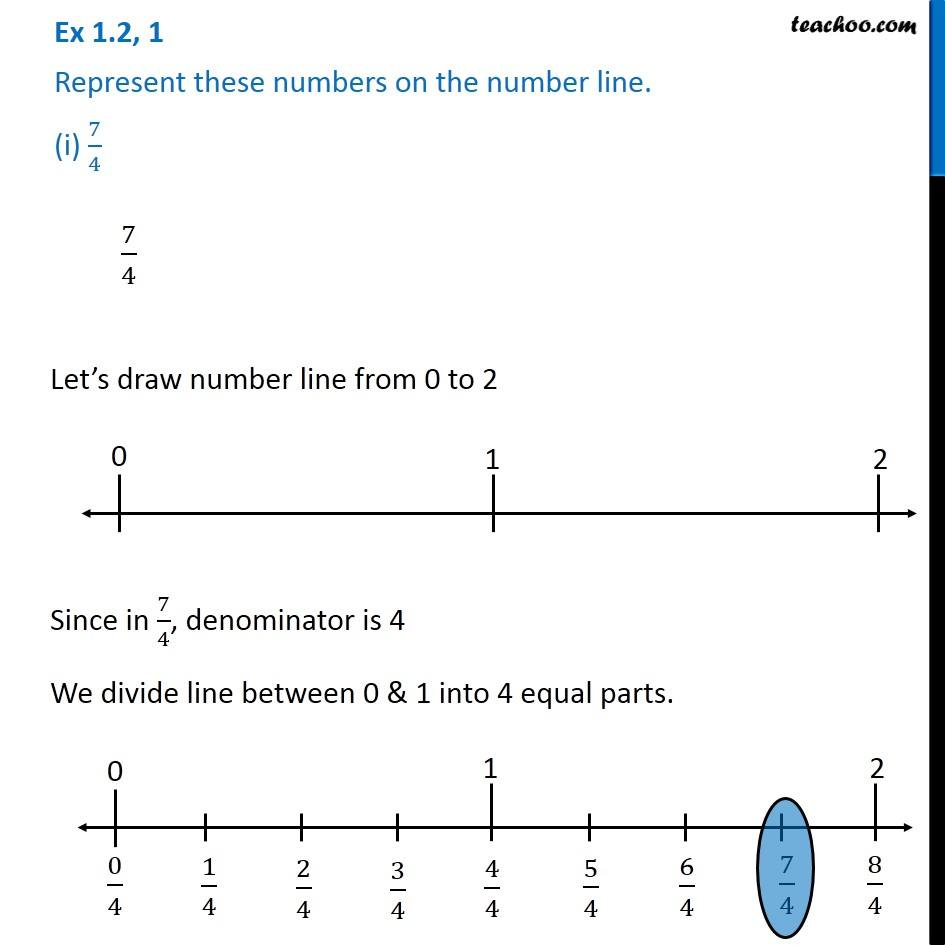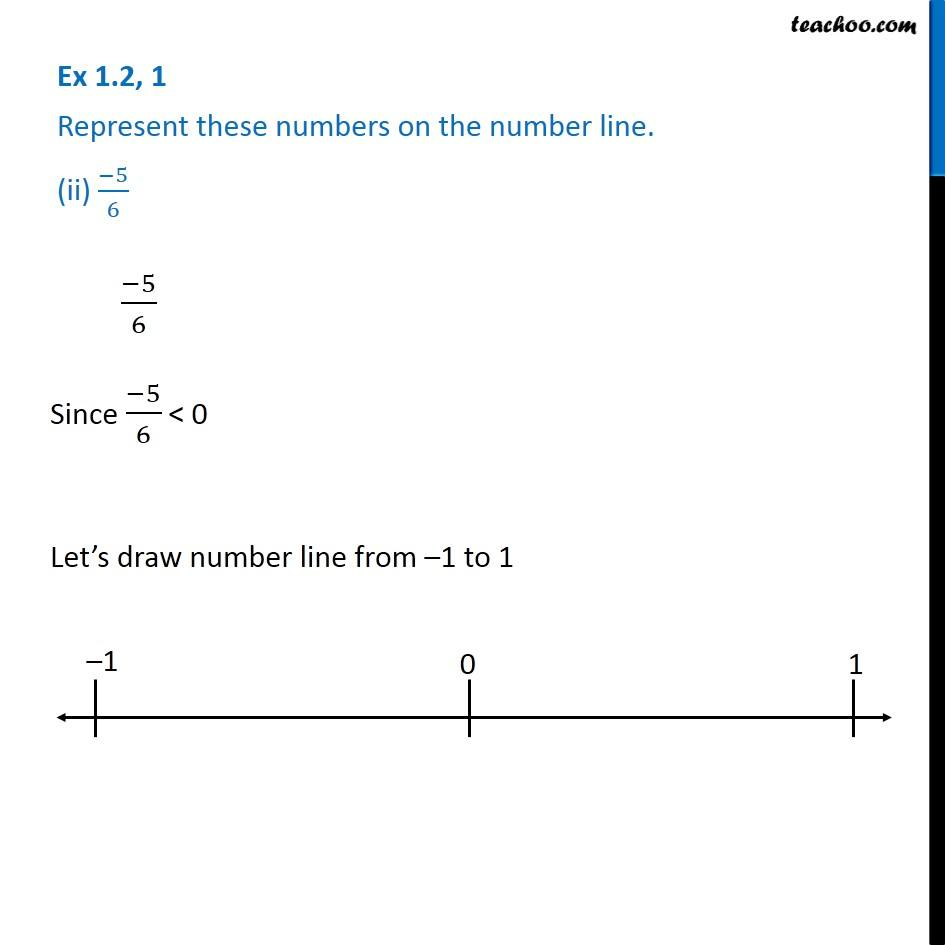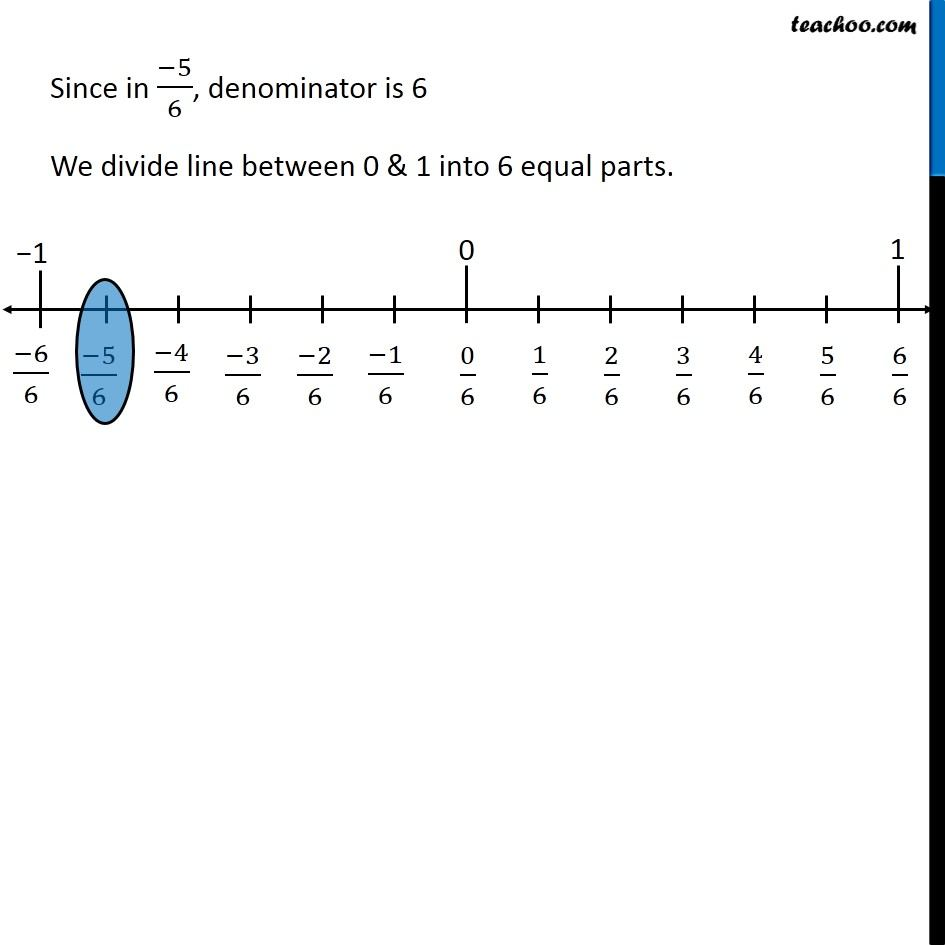Representing rational numbers on number line

Chapter 1 Class 8 Rational Numbers
Serial order wiseLearn in your speed, with individual attention - Teachoo Maths 1-on-1 Class

### Transcript

Question 1 Represent these numbers on the number line. (i) 7/4 7/4 Let s draw number line from 0 to 2 Since in 7/4, denominator is 4 We divide line between 0 & 1 into 4 equal parts. Question 1 Represent these numbers on the number line. (ii) ( 5)/6 ( 5)/6 Since ( 5)/6 < 0 Let s draw number line from 1 to 1 Since in ( 5)/6, denominator is 6 We divide line between 0 & 1 into 6 equal parts.